## General Mechanical

#### A transient analysis coupled with a static analysis in Workbench, Mechanical application

•CassioEM
Subscriber

I am trying to import the constraint distribution from my static analysis in order to use as a initial condition in my transient analysis.

The problem is when I export it, there are three option and all of them are not the constraint distribution. What am I doing wrong?

Can someone help me?

•jj77
Subscriber

It is not clear what you mean here - can you explain more (what do you mean with constrain distribution).

Also some images of what you are doing would help us understand.

•CassioEM
Subscriber

When I connect the static results to the transient setup, there are 4 options to import:

• Temperature;

• Imported cut boundary remote force;

• Imported cut boundary remote constraint;

• Imported cut boundary constraint.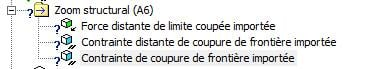The option that is closer to the constraint distribution is the last one. But the program specifies as displacements and not constraints.

How can I obtain the constraint distribution from the static analysis?

•CassioEM
Subscriber

I am working in a model in a transient analysis divided in two steps: the first one is the stabilization considering the mass, and the second is when the model is analyzed when it is applied variable forces.

The idea is to use the results from a static analysis to reduce the time of the first step. So I modeled a simple system and I simulated in the static mode.

Now I am trying to import the results to the transient analysis to start the simulation with the static results.

The simplified model: It is a beam connected with a spring and with a fixed support in the other extremity.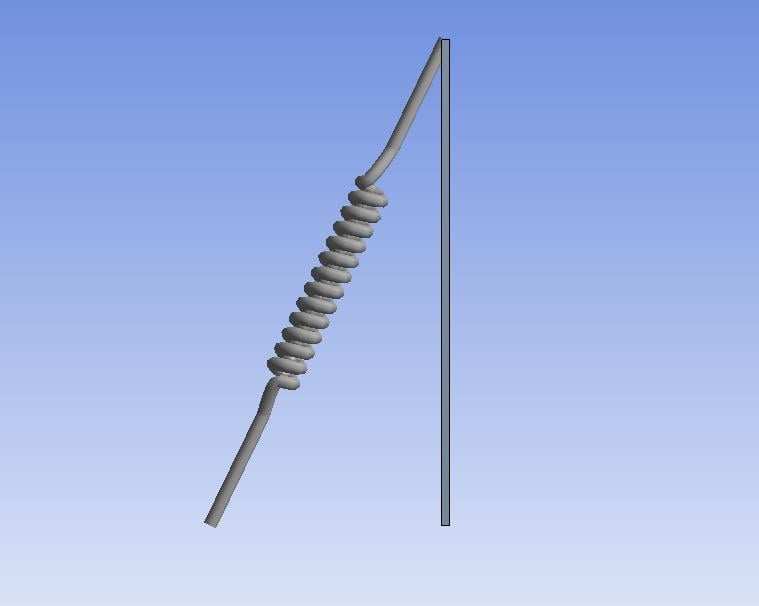The result obtained in the static analysis ( displacement distribution):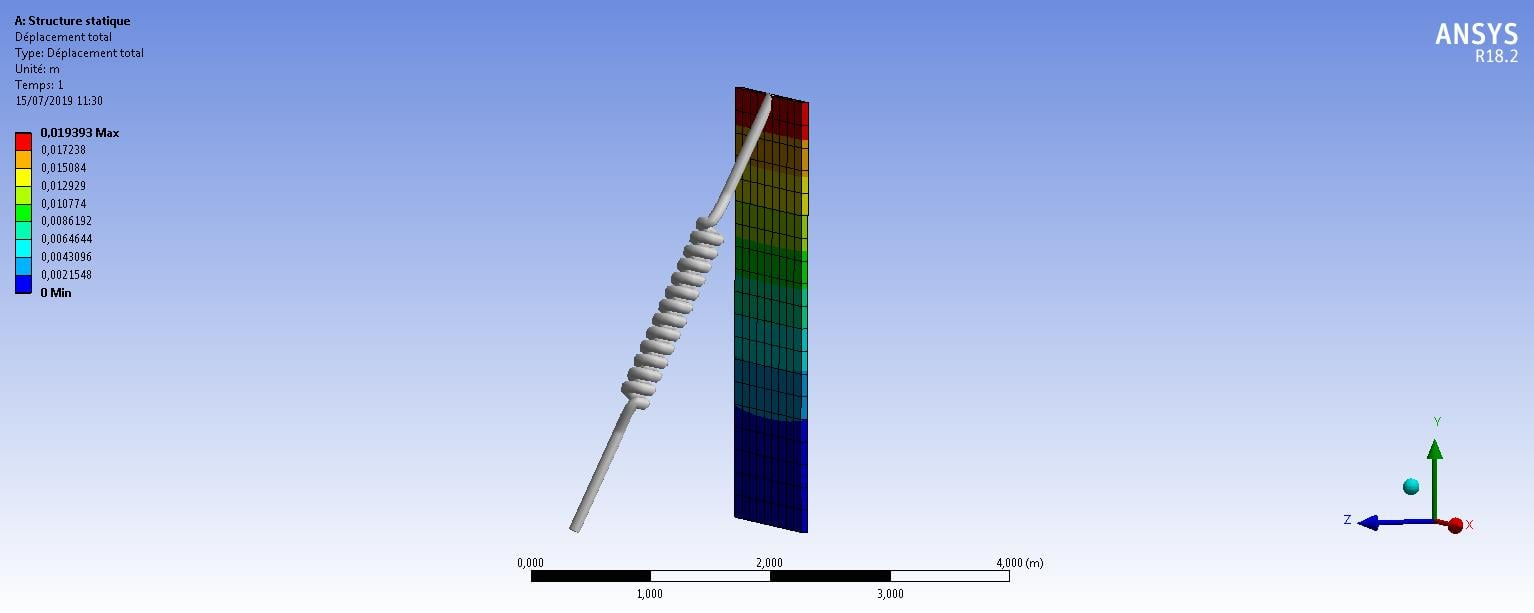And when I tried to import in the transient analysis, I don't know how can I define it in order to use as the initial situation.

Just to illustrate what I mean, I divided the transient simulation in two steps and in the second step, I defined a force applied in the tip just to tested it. The problem is the result is the same as the static mode, without changing with the force.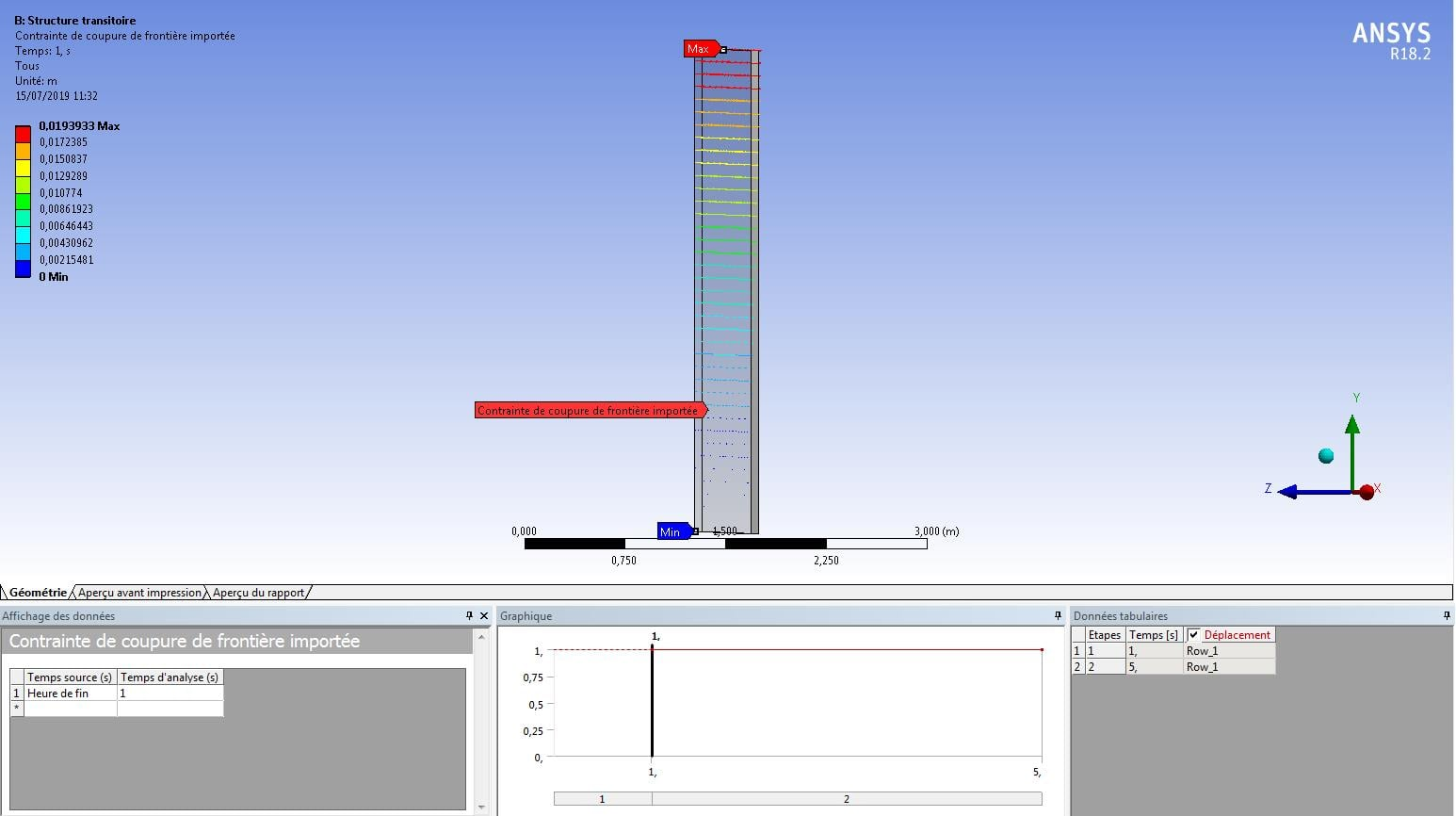Here the result showing a constant displacement. But I applied a force in the second step and it does not make sense.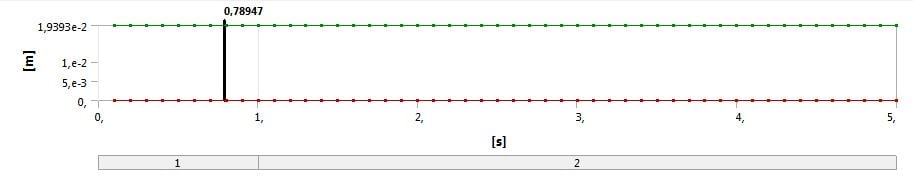I am thinking that I did something wrong when I imported the displacement distribution from the static analysis, but I don't know what.

Maybe it is something related to the time definition, but I don't know what I can change.

•jj77
Subscriber

What are you trying to do/analyse?

•CassioEM
Subscriber
I am trying to analyse a model under some dynamic conditions, like a variable force and variable displacement in some parts, for example.

The idea of the static analysis is just to reduce the time to stabilize the system in the first step of the transient simulation.
•jj77
Subscriber

Ok. Just have 3 steps and say two loads (one static one dynamic).

Steps:

1. The static load is on here (dynamic is 0), with no time integration effects which means it is a quasi static analysis.

2. Keep that force just to make sure that nothing happens (time integration set to on now).

3. Finally add the dynamic load and keep the static constant, and remember to use time integration effects again (as in step 2) on, to account for inertia and dynamics..

This is all done in transient, so no need to include static. Time integration effects are chosen under analysis settings and the time step definitions/step controls.

•CassioEM
Subscriber

I have a question related to the quasi static analysis: Which parameters should I considerer to determinate the time step?

Because for the transient analysis, I considered the time step as 0,0025 s because I have some phenomena which occur in a maximum frequency of 20Hz.

But a quasi static analysis should need less, right? I mean, considering that it is almost static, the time step should be bigger, right?

How can I precise the time step in a quasi static analysis?

•peteroznewman
Subscriber

Here is an example showing how the first two steps with time integration OFF, allow a static prestress to setup the third step with time integration ON to begin the Transient portion.

Any time increment will do for step 1 and 2. It doesn't affect much except what the "start" time is for step 3.

•jj77
Subscriber

Correct say if your total quasi static time is 1 s then a couple of steps are enough - so a time step of 0.1 s is more than enough.

•CassioEM
Subscriber

Thank you! I am working in my simulation and I will try the approach suggested!